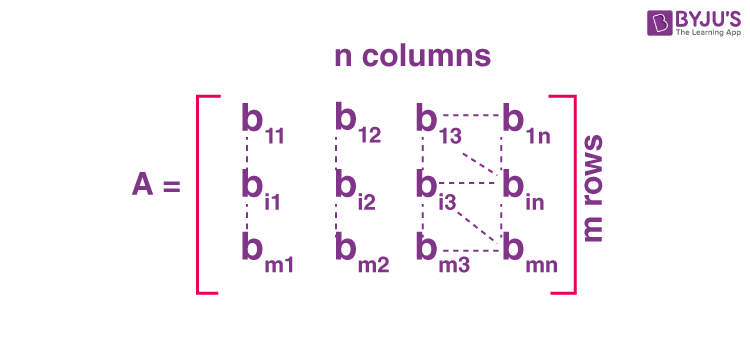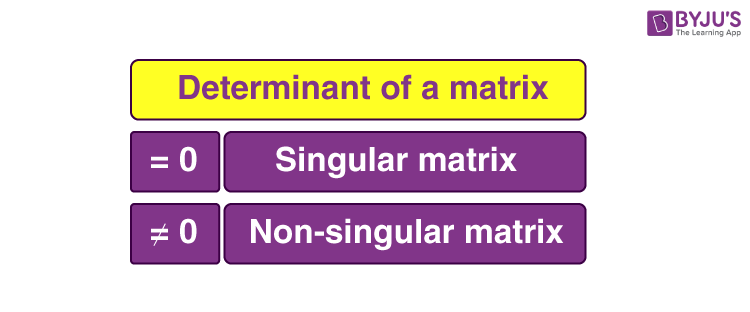# Singular Matrix

A matrix is an ordered arrangement of rectangular arrays of function or numbers, that are written in between the square brackets. Each row and column include the values or the expressions that are called elements or entries. The total number of rows by the number of columns describes the size or dimension of a matrix. The matrix representation is as shown below.The matrix shown above has m-rows (horizontal rows) and n-columns ( vertical column). The order of the matrix is given as m

$$\begin{array}{l}\times\end{array}$$
n.

## Types of matrices

We have different types of matrices in Maths, such as:

• Row matrix
• Column matrix
• Identity matrix
• Square matrix
• Rectangular matrix
• Singular Matrix

## What is Singular Matrix?

A square matrix (m = n) that is not invertible is called singular or degenerate. A square matrix is singular if and only if its determinant is 0.

If we assume that,

A and B are two matrices of the order, n x n satisfying the following condition:

AB = I = BA

Where I denote the identity matrix whose order is n.

Then, matrix B is called the inverse of matrix A.

Therefore, A is known as a non-singular matrix.The matrix which does not satisfy the above condition is called a singular matrix i.e. a matrix whose inverse does not exist.

## Determinant

Every square matrix has a determinant. The determinant is a mathematical concept that has a vital role in finding the solution as well as analysis of linear equations.

Suppose A is a matrix as given below:

$$\begin{array}{l}\large A = \begin{bmatrix} a & b & c\\ d & e & f\\ g & h & i \end{bmatrix}\end{array}$$

The determinant of the matrix A is denoted by |A|, such that;

$$\begin{array}{l}\large \begin{vmatrix} A \end{vmatrix} = \begin{vmatrix} a & b & c\\ d & e & f\\ g & h & i \end{vmatrix}\end{array}$$

The determinant can be calculated as:

$$\begin{array}{l}\large \begin{vmatrix} A \end{vmatrix} = a(ei – fh) – b(di – gf) + c (dh – eg)\end{array}$$

For a Singular matrix, the determinant value has to be equal to 0, i.e. |A| = 0.

Thus, a(ei – fh) – b(di – fg) + c(dh – eg) = 0

Example: Determine whether the given matrix is a Singular matrix or not

$$\begin{array}{l}\mathbf{\begin{bmatrix} 2 & 4 & 6\\ 2 & 0 & 2 \\ 6 & 8 & 14 \end{bmatrix}}\end{array}$$
.

Solution: Given

$$\begin{array}{l} \begin{bmatrix} 2 & 4 & 6\\ 2 & 0 & 2 \\ 6 & 8 & 14 \end{bmatrix}\end{array}$$

The Determinant is given by-

$$\begin{array}{l} 2(0 – 16) – 4 (28 – 12) + 6 (16 – 0) = -2(16) + 2 (16) = 0\end{array}$$

As the determinant is equal to 0, hence it is a Singular Matrix.

We already know that for a Singular matrix, the inverse of a matrix does not exist. Let us learn why the inverse does not exist.

The inverse of a matrix ‘A’ is given as-

$$\begin{array}{l}\mathbf{A’ = \frac{adjoint (A)}{\begin{vmatrix} A \end{vmatrix}}}\end{array}$$

for a singular matrix

$$\begin{array}{l}\begin{vmatrix} A \end{vmatrix} = 0\end{array}$$

the denominator term needs to be 0 for a singular matrix, that is not-defined.

Therefore, the inverse of a Singular matrix does not exist.

### Properties

Some of the important properties of a singular matrix are listed below:

• The determinant of a singular matrix is zero
• A non-invertible matrix is referred to as singular matrix, i.e. when the determinant of a matrix is zero, we cannot find its inverse
• Singular matrix is defined only for square matrices
• There will be no multiplicative inverse for this matrix

Visit BYJU’S to explore more about Matrix, Matrix Operation, and its application.